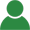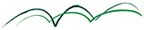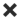ErrorHome Courses Course Calendar Teaching Team About Us Resources Contact
Course Calendar

# Managing Model Risk for Quants, Traders and Validators

## Day One

### Model risk and model validation outlook

• Managing model risk: value-based approach vs price-based approach. The credit crunch example
• Suggestions from model risk management in science. The real black swans
• Measuring model uncertainty. Practical meaning of no-arbitrage pricing and model completeness in liquid and illiquid markets
• Accounting standards (IAS/IFRS). Implications of fair value on model validation. Practical analysis of level 2 and 3 pricing. A case study on swaps and basis risk
• Market regulators: BIS, FSA, FED on stress testing, model risk and model validation. What changes after the crisis. Basel new principles
• From theory to practice: step-by-step building of a practical framework for model validation and the management of model risk

### Comparing and using alternative models

• Model comparison methodology
• Calibration and realism assessment
• How to use alternative models to quantify model risk
• First example: gap risk computation in leveraged notes. Structurals vs reduced-form models and their hidden consequences on gap losses
• Second example: local vs stochastic volatility models on time-dependent equity derivatives. Smile dynamics
• Third example: BGM vs short rate models for Interest Rate American-Bermudan. Hidden role of correlations and common misunderstandings

### Stress-testing design and pitfalls

• Stress testing models and stress testing with models (scenario analysis for portfolios)
• What to test? Stressing model assumptions and stressing model implementation (approximations, analytics and numerics)
• First Example: stress-tests using market information. Improving correlation skew modelling for efficient portfolio scenario analysis
• Second Example: stress scenarios design using historical information. Validating and improving mapping for bespoke credit portfolios
• Third Example: stress-testing pitfalls. Detect copulas' weaknesses for forward correlations and improve on them

## Day Two

### Understanding model evolution to prevent model losses

• Bringing hidden model assumptions to light and monitoring when they break down
• Example 1: how interest-rate consensus model broke down when the basis spreads exploded. How to find an analytical model that explains the new market. Consequences for term-structure building
• Example 2: modelling liquidity risk and liquidity charges. Funding liquidity and interactions with credit and discounting. Market liquidity and bid-ask. How changes in market fundamentals can shake the foundations of pricing

### Hedging Analysis and P&L Analysis

• Limits of pricing models when applied to hedging. How models are modified for efficient hedging. Validation of a real hedging strategy
• Practical example: Local volatility models vs stochastic volatility models in options hedging. The case of SABR and the shadow delta for the swaptions smile
• What we can get from P&L analysis and the delusions about it. The effect of model recalibration. The real cost of hedging and the charging of hedging costs

### Correlation

• The risk of wrong correlation assumptions and technical difficulties in modelling interdependencies
• Three tools to overcome technical difficulties. Examples: FX correlations, interest rate correlation parameterisations, correlation of stochastic volatility with the underlying
• Controlling model dimensionality and correlation rank
• Wrong correlation assumptions. 1-correlation risk with example on multifactor models. 0-correlation risk with example on counterparty risk. Correlation vs dependency

### Calibration

• Assessing calibration stability through comparison with market variability and analysis of the implied evolution of term structure of volatilities
• The effect of calibration instability on hedging
• Practical examples in dynamic hedging and pricing of American options
• Reverse engineering of counterparty quotes, consensus platforms, collateral regulations. Examples

MatLab workshop

## Day Three

### Approximations

• Validating an approximation. The operative steps. Monitoring market features that affect the reliability of approximations. Setting quantitative triggers
• Validation against Monte Carlo methods. Methodology. Examples from interest rates: the swaption approximation and the convexity adjustments for CMS
• Validating against analytic methods. SABR approximation vs the SABR model

### Extrapolations

• Interpolation and extrapolations. Dangers of extrapolations and how to avoid them
• Turning extrapolation into interpolation by adding data: example from volatility smile
• Turning extrapolation into interpolation by changing variable: example from correlation skew

### Arbitrage

• The practical meaning of arbitrage trading and statistical arbitrage. Risks of hedge funds and proprietary desks. Models' limitations in detecting arbitrage
• Analysing and validating arbitrage strategies by revealing their nature of directional trades on market uncertainty
• Practical example on the Equity/Bond capital-structure arbitrage
• Cap-swaption arbitrage and how it broke down

### Payoff Errors

• Last but not least: are we sure the payoff is right? Mathematical errors and legal errors
• Examples of payoff errors widely common in the market: index options and bilateral counterparty risk

A final synthesis with practical MatLab workshops on model risk and model validation